• +91 9971497814
• info@interviewmaterial.com

# Polynomials Ex-2.4 Interview Questions Answers

### Related Subjects

Question 1 :

Verify that the numbers given alongside of thecubic polynomials below are their zeroes. Also verify the relationship betweenthe zeroes and the coefficients in each case:

(i) 2x3+x2-5x+2; -1/2,1, -2               (ii) x3-4x2+5x-2 ;2, 1, 1

(i) 2x3+x2-5x+2; -1/2,1, -2

Solution:

Given, p(x) 2x3+x2-5x+2

And zeroes for p(x) are = 1/2, 1, -2

p(1/2) = 2(1/2)3+(1/2)2-5(1/2)+2

= (1/4)+(1/4)-(5/2)+2

= 0

p(1)= 2(1)3+(1)2-5(1)+2

= 0

p(-2)= 2(-2)3+(-2)2-5(-2)+2

= 0

Hence, proved 1/2, 1, -2 are the zeroes of 2x3+x2-5x+2.

Now, comparing the given polynomial withgeneral expression, we get;

ax3+bx2+cx+d

= 2x3+x2-5x+2

a=2, b=1, c= -5 and d = 2

As we know, if α, β, γ are the zeroes of thecubic polynomial ax3+bx2+cx+d , then;

α +β+γ = –b/a

αβ+βγ+γα = c/a

α βγ = – d/a.

Therefore, putting the values of zeroes of thepolynomial,

α+β+γ = ½+1+(-2)

= -1/2

= –b/a

αβ+βγ+γα = (1/2×1)+(1 ×-2)+(-2×1/2)

= -5/2

= c/a

α β γ = ½×1×(-2)

= -2/2

= -d/a

Hence, the relationship between the zeroes andthe coefficients are satisfied.

(ii) x3-4x2+5x-2 ;2, 1, 1

Solution:

Given, p(x) = x3-4x2+5x-2

And zeroes for p(x) are 2,1,1.

p(2)= 23-4(2)2+5(2)-2

= 0

p(1) = 13-(4×1)+(5×1)-2

= 0

Hence proved, 2, 1, 1 are the zeroes of x3-4x2+5x-2

Now, comparing the given polynomial withgeneral expression, we get;

ax3+bx2+cx+d = x3-4x2+5x-2

a = 1, b = -4, c = 5 and d = -2

As we know, if α, β, γ are the zeroes of thecubic polynomial ax3+bx2+cx+d , then;

α + β + γ = –b/a

αβ + βγ + γα = c/a

α β γ = – d/a.

Therefore, putting the values of zeroes of thepolynomial,

α +β+γ = 2+1+1 = 4 = -(-4)/1 = –b/a

αβ+βγ+γα = 2×1+1×1+1×2 = 5 = 5/1= c/a

αβγ = 2×1×1 = 2 = -(-2)/1 = -d/a

Hence, the relationship between the zeroes andthe coefficients are satisfied.

Question 2 :

If the zeroes of the polynomial x3-3x2+x+1 are a – b, a, a + b, find a and b.

Solution:

We are given with the polynomial here,

p(x) = x3-3x2+x+1

And zeroes are given as a – b, a, a + b

Now, comparing the given polynomial withgeneral expression, we get;

px3+qx2+rx+s = x3-3x2+x+1

p = 1, q = -3, r = 1 and s = 1

Sum of zeroes = a – b + a + a + b

-q/p = 3a

Putting the values q and p.

-(-3)/1 = 3a

a=1

Thus, the zeroes are 1-b, 1, 1+b.

Now, product of zeroes = 1(1-b)(1+b)

-s/p = 1-b2

-1/1 = 1-b2

b2 = 1+1 = 2

b = √2

Hence,1-√2, 1 ,1+√2 are the zeroes of x3-3x2+x+1.

Question 3 : two zeroes of the polynomial x4-6x3-26x2+138x-35 are 2 ±3, findother zeroes.

Answer 3 :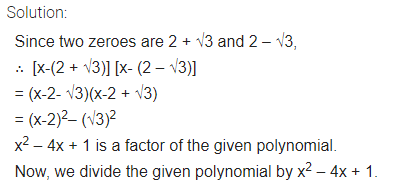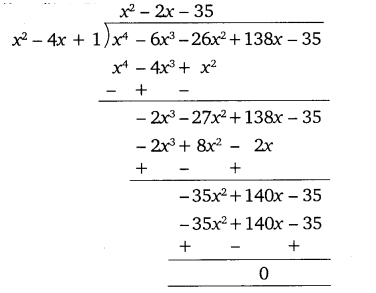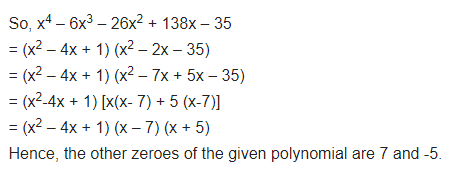Question 4 :

_If the polynomial x4 – 6x3 + 16x2 – 25x + 10 is divided by another polynomial x2 – 2x + k, the remainder comes out to be x +a, find k and a.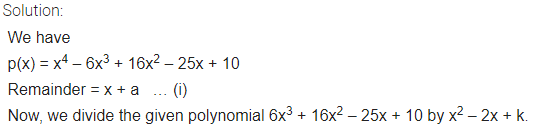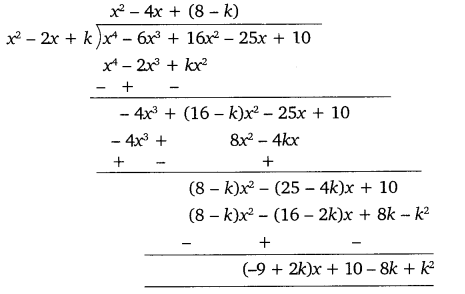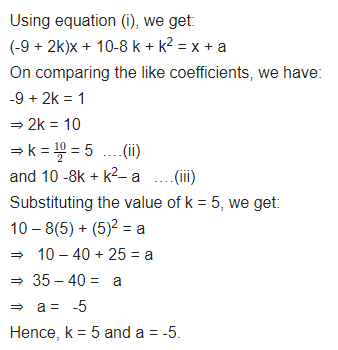Todays Deals### Polynomials Ex-2.4 Contributorskrishan

Name:
Email:

# Latest News# 9000 interview questions in different categories